Some time ago, I have encountered a programming exercise in which the goal was to implement Luhn algorithm for credit card number validation. At first I implemented the algorithm in Ruby and then decided to implement it in Elixir. Eventually, I like how Elixir version looks like.

# Initial version

It is assumed that credit card numbers are being read from a file and that check digit is included in a credit card number.

The Luhn algorithm is quite straightforward. Description from Wikipedia 1:

The formula verifies a number against its included check digit, which is usually appended to a partial account number to generate the full account number. This number must pass the following test:

From the rightmost digit, which is the check digit, moving left, double the value of every second digit; if the product of this doubling operation is greater than 9 (e.g., 8 × 2 = 16), then sum the digits of the products (e.g., 16: 1 + 6 = 7, 18: 1 + 8 = 9). Take the sum of all the digits. If the total modulo 10 is equal to 0 (if the total ends in zero) then the number is valid according to the Luhn formula; else it is not valid.

My main function of the Luhn algorithm clearly outlines the steps of the algorithm:

``````  def check_validity(line) do
String.strip(line)
|> remove_whitespace
|> String.reverse
|> double_every_second_digit
|> sum_double_numbers
|> sum_digits
|> modulo
|> is_valid?
end``````
Function `String.strip(line)` is used to remove any newline codes. Function `remove_whitespace/1` is used to format credit card numbers from “1234 5678 4321 8765” to 1234567843218765".

``````  def remove_whitespace(string) do
String.codepoints(string)
|> Enum.reject(fn(x) -> x == " " end)
|> List.to_string
end
``````

The part that made me think hard is doubling of every second digit. It was implemented by converting a string with a credit card number into list of codepoints and converting this list into list of lists of at most 2 elements, doubling every second element in each list and then those lists were flattened into one list:

``````  def double_every_second_digit(string) do
String.codepoints(string)
|> Stream.chunk(2, 2, [])
|> Enum.reduce([], fn(x, acc) -> acc ++ double_second_element(x) end)
|> List.flatten
end

defp double_second_element([a]),  do: [a]
defp double_second_element([a,b]) do
[a, (String.to_integer(b) * 2) |> Integer.to_string]
end
``````

Function `sum_double_numbers/1` converts each number from a list of numbers (eg., `["2", "18"]`) into list of codepoints. If this list contains two codepoints, then it sums them, otherwise the codepoint is returned as is.

``````  def sum_double_numbers(nums) do
Enum.map(nums, fn(x) -> String.codepoints(x) |> sum_double_number end)
end

defp sum_double_number([a]), do: a
defp sum_double_number([a, b]) do
String.to_integer(a) + String.to_integer(b)
|> Integer.to_string
end
``````

Lastly, function `is_valid?/1` returns “1” if a number is valid and “0” if it is invalid.

Initial version code is available at Gitlab: Luhn algorithm v1

# Refactoring

To search for possible improvements for the code, I have installed a static code analysis tool called credo. After running `mix credo` in my project I have got 8 refactoring opportunities and 1 code readability issue: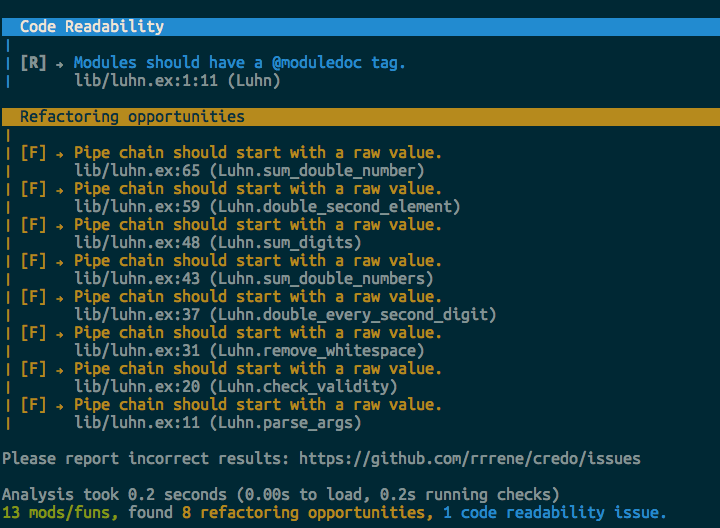This means that I should refactor my pipes to start with a raw value (clearly!). For example, function `remove_whitespace/1` will looks like:

``````  def remove_whitespace(string) do
string
|> String.codepoints
|> Enum.reject(fn(x) -> x == " " end)
|> List.to_string
end
``````

#### Modules should have a @moduledoc tag

Credo suggests me to add @moduledoc tag and write some documentation for Luhn module:

``````defmodule Luhn do
@moduledoc """
Implements Luhn algorithm for credit card number validation.

## Examples

iex> Luhn.check_validity("1556 9144 6285 339")
"1"
"""
``````

# Benchmarking

In order to benchmark my code I have used tool Benchfella.

Very simple script has been made to be used with Benchfella:

``````defmodule LuhnBench do
use Benchfella

bench "card number validity" do
Luhn.check_validity "6370 1675 9034 6211 774"
end
end
``````

Running this script gave results in 30-34 µs/op (with 50000 iterations).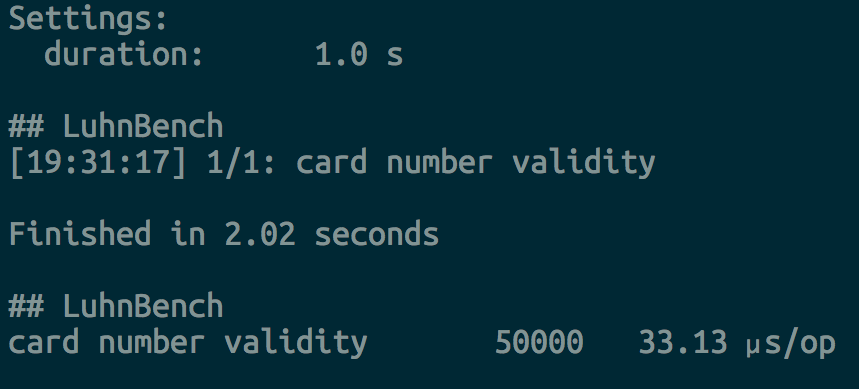## Improving performance

On elixir slack chat Nathan Youngman gave suggestions about improving functions `remove_whitespace/1` and `double_every_second_digit/1`.

Function `remove_whitespace/1` can be implemented using `String.replace/4`:

``````  def remove_whitespace(string) do
String.replace(string, " ", "")
end
``````

Comparison of both implementations shows a significant improvement when `String.replace/4` is used: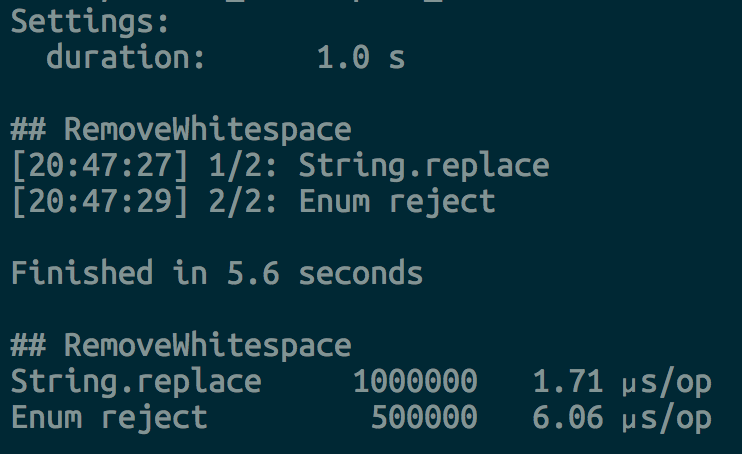Function `double_every_second_digit/1` could be implemented using map with indexes and the card number could be represented as integer:

``````  def double_every_second_digit(number) do
number
|> Integer.digits
|> Enum.with_index
|> Enum.map(&double_digit(&1))
end

defp double_digit({digit, index}) when Integer.is_odd(index) do
digit * 2
end
defp double_digit({digit, _}), do: digit
``````

Again it shows improvement over the version with lists: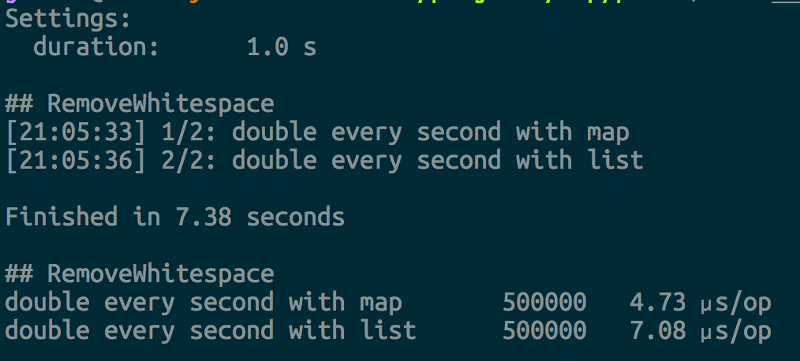As a general suggestion that can be derived from this optimization is that it is beneficial to pass credit card number as an integer instead of a string. By refactoring code to pass around credit card numbers as integers we again see notable improvement in speed: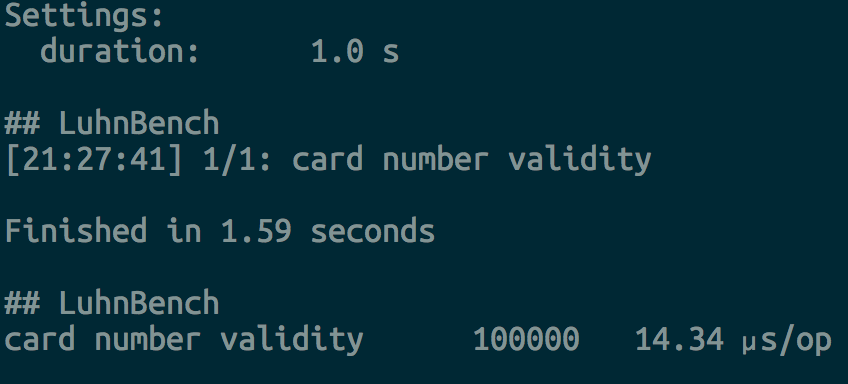We went from 33,13 µs/op (with 50000 iterations) to 14,34 µs/op (with 100000 iterations).

# Conclusion

We implemented Luhn algorithm in Elixir and saw how pipelines outline steps of the algorithm. After coding the initial version we used credo to receive suggestions on style of our code. Lastly, we improved performance of the algorithm by implementing several functions differently and by treating a credit card number as an integer instead of a string. Nice side-effect of this refactoring is that credo does not find any problems with the code.

Latest source code can be found on Gitlab: Luhn algorithm